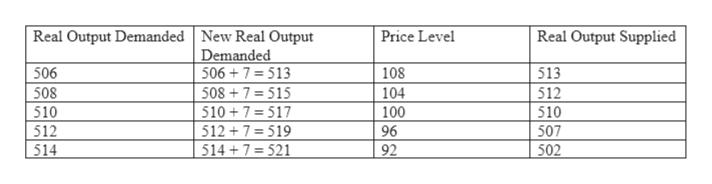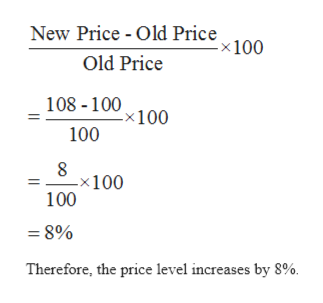# Refer to the table below.Real Output Demanded, BillionsPrice Level Real Output Supplied, Billions\$ 506108\$ 5135081045125101005105129650751492502Instructions: Enter your anwers as whole numbers. A). What is the equilibrium level of output? What is the equilibrium price level? B). Suppose that aggregate demand increases such that the amount of real output demanded rises by \$ 7 billion at each price level. Insert the new values for real output demanded in the table below.Real Output Demanded, BillionsNew Real Output Demanded, BillionsPrice LevelReal Output Supplied, Billions\$ 506 108\$ 513508 104512510 100510512 96507514 92502What is the new equilibrium level of output? What is the new equilibrium price level? By what percentage will the price level increase? Will this inflation be demand-pull inflation or will it be cost-push inflation?C) If potential real GDP ( that is, full-employment GDP) is \$ 510 billion, what will be the size of the positive GDP gap after the change in aggregate demand?If government wants to use fiscal policy to counter the resulting inflation without changing tax rates, would it increase governmnet spending or decrease it?

Question

Refer to the table below.

 Real Output Demanded, Billions Price Level Real Output Supplied, Billions \$ 506 108 \$ 513 508 104 512 510 100 510 512 96 507 514 92 502

Instructions: Enter your anwers as whole numbers.

A). What is the equilibrium level of output? What is the equilibrium price level?

B). Suppose that aggregate demand increases such that the amount of real output demanded rises by \$ 7 billion at each price level. Insert the new values for real output demanded in the table below.

 Real Output Demanded, Billions New Real Output Demanded, Billions Price Level Real Output Supplied, Billions \$ 506 108 \$ 513 508 104 512 510 100 510 512 96 507 514 92 502

What is the new equilibrium level of output?

What is the new equilibrium price level?

By what percentage will the price level increase?

Will this inflation be demand-pull inflation or will it be cost-push inflation?

C) If potential real GDP ( that is, full-employment GDP) is \$ 510 billion, what will be the size of the positive GDP gap after the change in aggregate demand?

If government wants to use fiscal policy to counter the resulting inflation without changing tax rates, would it increase governmnet spending or decrease it?

check_circle

star
star
star
star
star
1 Rating
Step 1

(a) Equilibrium exists when the quantity demanded is equal to the quantity supplied. At equilibrium, there is no surplus or shortage in the market.  As shown in the table, quantity demanded is equal to the quantity supplied at price level of \$100. So, the equilibrium price is \$100 and the equilibrium quantity is 510 billion.

Step 2

(b) The table below shows the new real output demanded:help_outlineImage TranscriptioncloseReal Output Demanded New Real Output Demanded 506+7=513 Real Output Supplied Price Level 506 508 510 512 108 513 508 7=515 510+7=517 512+7=519 514+7 = 521 104 512 510 100 96 507 514 92 502 fullscreen
Step 3

The new real output demanded is equal to the real output supplied at price level \$108.

So, the new equilibrium price is 108 and the new equ...help_outlineImage TranscriptioncloseNew Price - Old Price x 100 Old Price 108 -100 -x100 100 -x100 100 =8% Therefore, the price level increases by 8% fullscreen

### Want to see the full answer?

See Solution

#### Want to see this answer and more?

Solutions are written by subject experts who are available 24/7. Questions are typically answered within 1 hour.*

See Solution
*Response times may vary by subject and question.
Tagged in